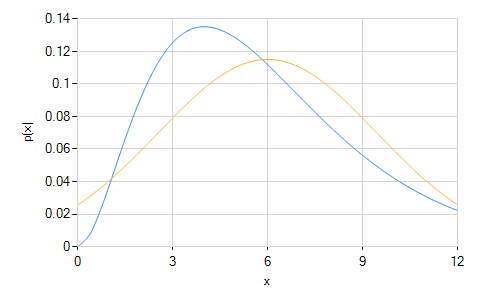﻿ChiSquaredDistribution Class# ChiSquaredDistribution Class

Represents a χ2 distribution.Inheritance Hierarchy

Namespace:  Meta.Numerics.Statistics.Distributions
Assembly:  Meta.Numerics (in Meta.Numerics.dll) Version: 4.1.4Syntax
`public sealed class ChiSquaredDistribution : ContinuousDistribution`

The ChiSquaredDistribution type exposes the following members.Constructors
NameDescriptionChiSquaredDistribution
Initializes a new χ2 distribution.
TopProperties
NameDescriptionDegreesOfFreedom
Gets the number of degrees of freedom ν of the distribution.ExcessKurtosis
Gets the excess kurtosis of the distribution.
(Overrides UnivariateDistributionExcessKurtosis.)Mean
Gets the mean of the distribution.
(Overrides UnivariateDistributionMean.)Median
Gets the median of the distribution.
(Overrides ContinuousDistributionMedian.)Skewness
Gets the skewness of the distribution.
(Overrides UnivariateDistributionSkewness.)StandardDeviation
Gets the standard deviation of the distribution.
(Inherited from UnivariateDistribution.)Support
Gets the interval over which the distribution is non-vanishing.
(Overrides ContinuousDistributionSupport.)Variance
Gets the variance of the distribution.
(Overrides UnivariateDistributionVariance.)
TopMethods
NameDescriptionCentralMoment
Computes a central moment of the distribution.
(Overrides ContinuousDistributionCentralMoment(Int32).)Cumulant
Computes a cumulant of the distribution.
(Overrides UnivariateDistributionCumulant(Int32).)Equals
Determines whether the specified object is equal to the current object.
(Inherited from Object.)ExpectationValue
Computes the expectation value of the given function.
(Inherited from ContinuousDistribution.)GetHashCode
Serves as the default hash function.
(Inherited from Object.)GetRandomValue
Generates a random variate.
(Inherited from ContinuousDistribution.)GetRandomValues
Generates the given number of random variates.
(Inherited from ContinuousDistribution.)GetType
Gets the Type of the current instance.
(Inherited from Object.)Hazard
Computes the hazard function.
(Inherited from ContinuousDistribution.)InverseLeftProbability
Returns the point at which the cumulative distribution function attains a given value.
(Overrides ContinuousDistributionInverseLeftProbability(Double).)InverseRightProbability
Returns the point at which the right probability function attains the given value.
(Inherited from ContinuousDistribution.)LeftProbability
Returns the cumulative probability to the left of (below) the given point.
(Overrides ContinuousDistributionLeftProbability(Double).)ProbabilityDensity
Returns the probability density at the given point.
(Overrides ContinuousDistributionProbabilityDensity(Double).)RawMoment
Computes a raw moment of the distribution.
(Overrides ContinuousDistributionRawMoment(Int32).)RightProbability
Returns the cumulative probability to the right of (above) the given point.
(Overrides ContinuousDistributionRightProbability(Double).)ToString
Returns a string that represents the current object.
(Inherited from Object.)
TopRemarks

A chi squared distribution is an asymmetrical distribution ranging from zero to infinity with a peak near its number of degrees of freedom ν. It is a one-parameter distribution determined entirely by the parameter ν.The figure above shows the χ2 distribution for ν = 6, as well as the normal distribution with equal mean and variance for reference.

The sum of the squares of ν independent standard-normal distributed variables is distributed as χ2 with ν degrees of freedom.The χ2 distribution appears in least-squares fitting as the distribution of the sum-of-squared-deviations under the null hypothesis that the model explains the data. For example, the goodness-of-fit statistic returned by the model our model fitting methods (FitToFunction(FuncDouble, T, Double, Double), FitToLinearFunction(FuncT, Double), FitToLine, and others) follows a χ2 distribution.See Also